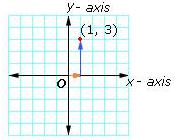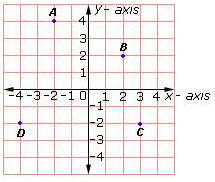Definition Of Y-Coordinate

The second number in an ordered pair is the y-coordinate.

Examples of Y Coordinate

In the ordered pair (2, 4), the y-coordinate is 4
In the ordered pair (1, - 7), the y-coordinate is - 7
To reach the point (1, 3), move 1 unit to the left from the origin and then 3 units upSolved Example on y-coordinate

Ques: Which of the points has 2 as the y-coordinate?A. A
B. B
C. C
D. D